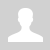Python. Nested loops
 11.1.2.7 use nested loops when solving problems 11.1.2.6 debug a program Python. Nested loops A nested loop has one loop inside of another.  These are typically used for working with two dimensions such as printing stars in rows and columns as shown below.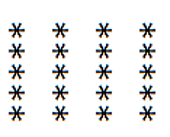When a loop is nested inside another loop, the inner loop runs many times inside the outer loop. In each iteration of the outer loop, the inner loop will be re-started. The inner loop must finish all of its iterations before the outer loop can continue to its next iteration. Example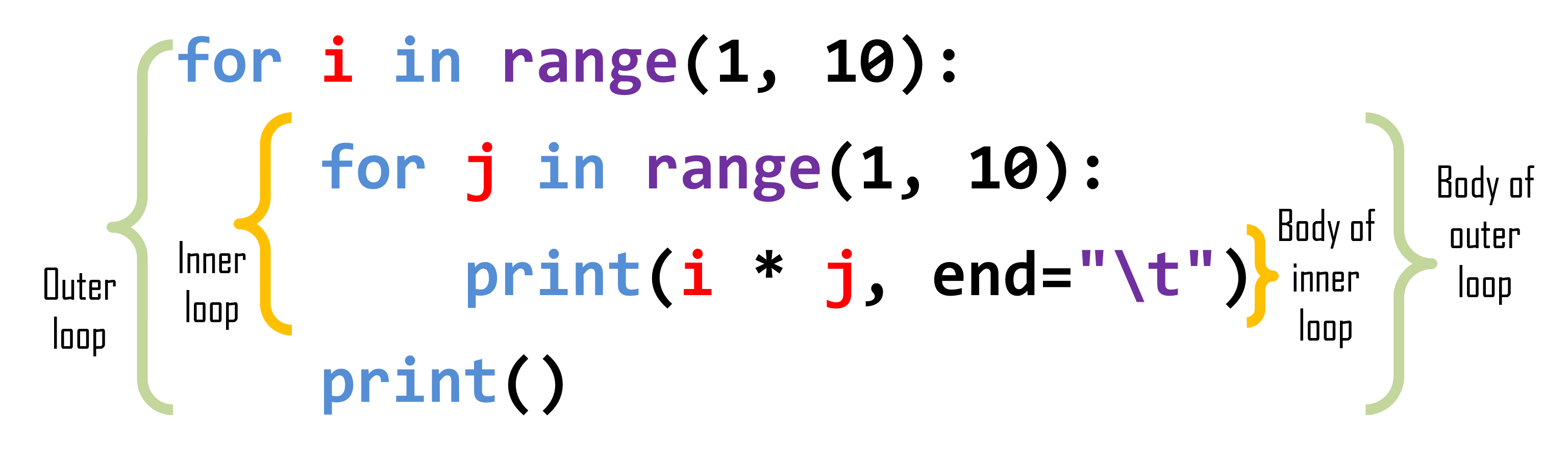Output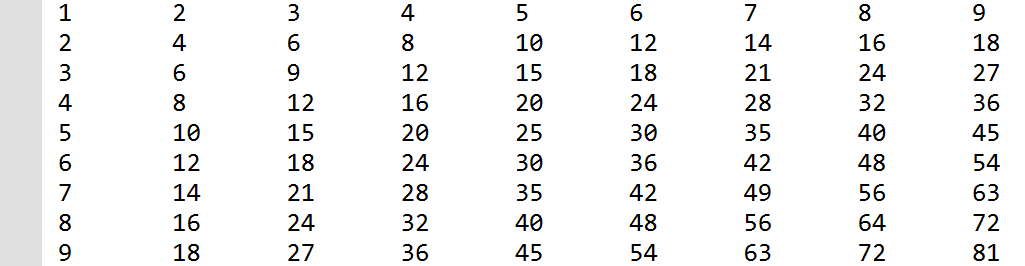Trace nested loops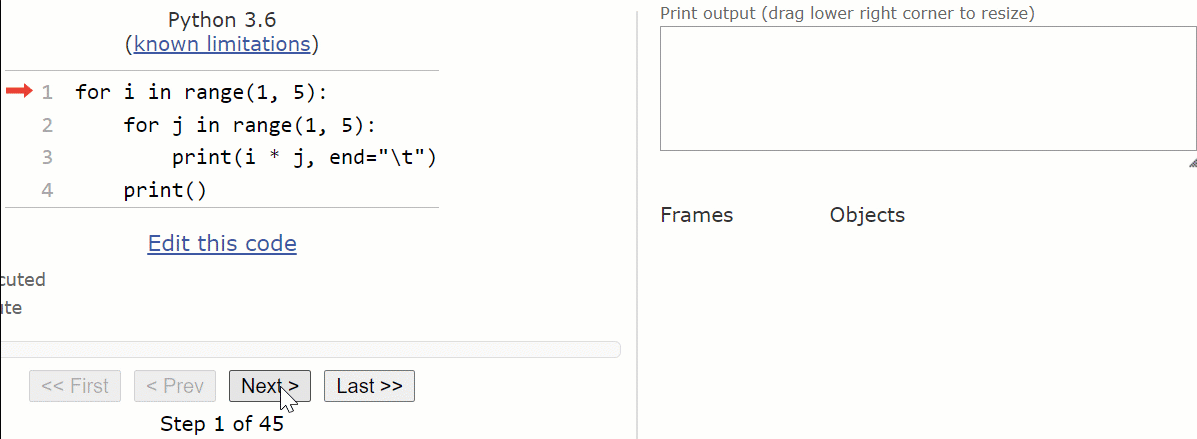Task 1. Output the next stars.```n = int(input()) m = int(input()) for i in range(n):      for j in range(m):   # nested loop         print('*', end='')     print()``` Task 2. Output multiplication table in line for number n. (n < 10) ```k = int(input()) for i in range(1, 10):     print(i, '*', k, '=', k * i, sep='', end='\t')``` Input: 5 Output: 1*5=5       2*5=10    3*5=15     4*5=20   5*5=25   6*5=30   7*5=35   8*5=40   9*5=45 Task 3. How to output a full multiplication table?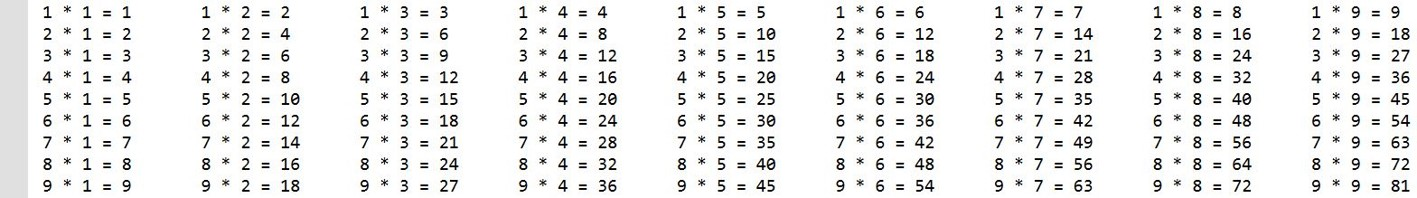```for i in range(1, 10):                      # start of outer loop             for j in range(1, 10):                  # start of inner loop         print(i, '*', j, '=', i * j, end='\t’) # output the multiplication table     print()                                       # newline``` Task 3. The second way of solving ```i = 1               # the initial value of the first factor while i < 10:       # condition for the first factor     j = 1           # the initial value of the second multiplier     while j < 10:   # the condition for the second multiplier          print(i, '*', j, '=', i * j, end='\t') # output         j += 1      # the change of the second multiplier in the inner loop     i += 1          # change the first multiplier in the outer loop     print()         # output new line``` Commands break and continue in nested loops ```while True:             # start of external loop     while True:         # the start of the inner loop           if [condition]:             continue    # the transition to the next iteration in the inner loop          if [condition]:             break       # the output of the inner loop       if [condition]:         continue        # the transition to the next iteration in the outer loop     if [condition]:         break           # the output of the outer loop``` Questions: Exercises: Ex. 1 Write a program to print the following start pattern using the for loop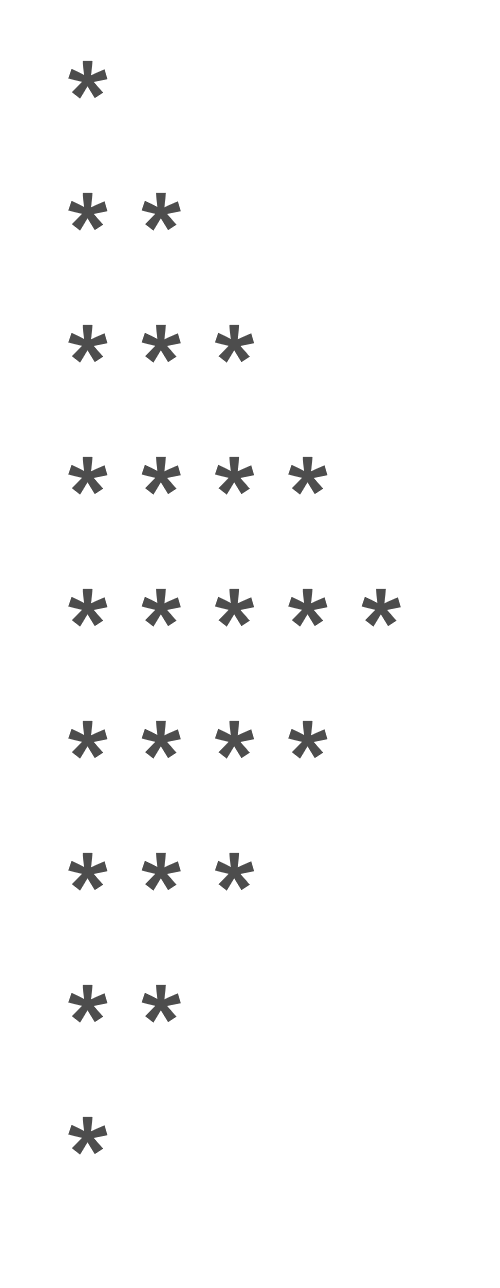Ex. 2 In number theory, a perfect number is a positive integer that is equal to the sum of its positive divisors, excluding the number itself. For instance, 6 has divisors 1, 2 and 3 (excluding itself), and 1 + 2 + 3 = 6, so 6 is a perfect number. Write a program to print all perfect numbers from 1 to 100 .u-star-rating-13 { list-style:none; margin:0px; padding:0px; width:65px; height:13px; position:relative; background: url('/.s/t/2001/rating13.png') top left repeat-x } .u-star-rating-13 li{ padding:0px; margin:0px; float:left } .u-star-rating-13 li a { display:block;width:13px;height: 13px;line-height:13px;text-decoration:none;text-indent:-9000px;z-index:20;position:absolute;padding: 0px;overflow:hidden } .u-star-rating-13 li a:hover { background: url('/.s/t/2001/rating13.png') left center;z-index:2;left:0px;border:none } .u-star-rating-13 a.u-one-star { left:0px } .u-star-rating-13 a.u-one-star:hover { width:13px } .u-star-rating-13 a.u-two-stars { left:13px } .u-star-rating-13 a.u-two-stars:hover { width:26px } .u-star-rating-13 a.u-three-stars { left:26px } .u-star-rating-13 a.u-three-stars:hover { width:39px } .u-star-rating-13 a.u-four-stars { left:39px } .u-star-rating-13 a.u-four-stars:hover { width:52px } .u-star-rating-13 a.u-five-stars { left:52px } .u-star-rating-13 a.u-five-stars:hover { width:65px } .u-star-rating-13 li.u-current-rating { top:0 !important; left:0 !important;margin:0 !important;padding:0 !important;outline:none;background: url('/.s/t/2001/rating13.png') left bottom;position: absolute;height:13px !important;line-height:13px !important;display:block;text-indent:-9000px;z-index:1 } Категория: Programming languages | Добавил: bzfar77 (14.10.2021) Просмотров: 60 | Теги: Loop, Python, nested, For | Рейтинг: 5.0/1
 Всего комментариев: 0